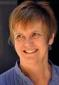# Simple question re Cholesky decomposition

3 posts / 0 newOffline
Joined: 02/04/2010 - 02:20
Simple question re Cholesky decomposition

I'm running a multivariate ACE model with raw data and matrices input, based on a version by Hermine Maes.
Changing the start value for the paths from .6 to .5 makes the c path estimates flip from positive to negative.
Am I right in assuming I can just ignore the sign, because the true estimate should be the (positive) square root of the squared path?Offline
Joined: 07/31/2009 - 15:14
Yes, in general in any factor

Yes, in general in any factor model if all the factor loadings change sign, the model is equivalent. It is also equivalent if the factor loadings in any number of columns of the factor loading matrix change sign. Note that while it is not generally necessary to "do" anything about it, bounding the first free element of a factor loading column to be non-negative does eliminate this source of indeterminacy. However if one were, for example, running bootstrap simulations, then it would be necessary to impose the constraints in order to stop the presence of both +ve and -ve sets of estimates causing inflated estimates of the bootstrap confidence intervals.Offline
Joined: 07/31/2009 - 15:24
As a much simpler followup to

As a much simpler followup to Mike N.'s comment: if the values in question are free parameters then specifying a lower bound of 0 will ensure they are positive. If the value are arbitrary cells of an algebra, then a mxConstraint() is needed. The point is to avoid using mxConstraint() if possible.